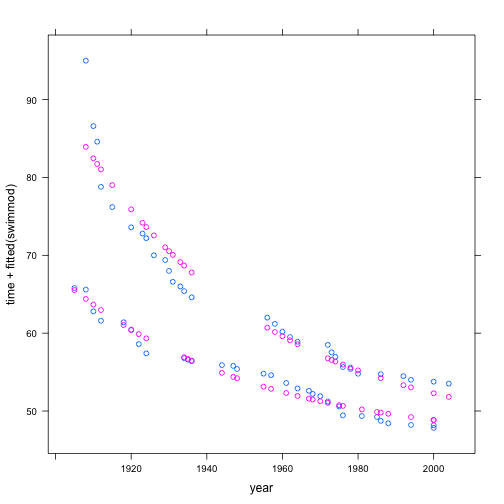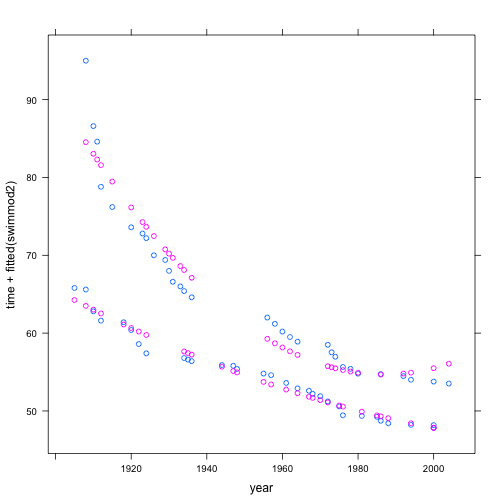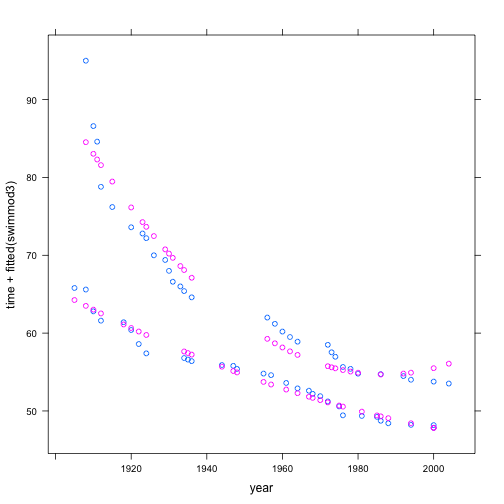# Stats 155 Class Notes 2012-09-24

## Form Groups for Car Project

Outline the used car project and show some data from http://www.cars.com. Perhaps MINI Coopers.

Get students to form groups right now, oriented around different kinds of cars. They should make a Google Spreadsheet and share it with all the other members of their group.

The statistical models we are studying account for the variation in a response variable using the variation in explanatory variables. Some points:

• We can quantify the variation in a quantitative variable by measuring the variance.
• Our statistical models take the form of a function, with the explanatory variables as inputs. The output corresponds to the response variable.
• We haven't yet encountered a good way to quantify the variation in categorical variables. What's more, the mathematics we have studied, e.g. calculus, doesn't provide us with a ready notation for a function that has a category as an output.
• Categories as inputs, however, are no problem. For instance, here is a function that takes an animal name as input and gives back a number as an input:
fetchData("m155development.R")  # load the software

## Retrieving from http://www.mosaic-web.org/go/datasets/m155development.R

##  TRUE

f = listFun(Antelope = 2, Beaver = -1, Cougar = 7)
f("Cougar")

## Cougar
##      7

• There is a standard modeling framework. There are many, many different ways in which you could combine inputs into an output. The one we will use is based in the mathematics of low-order polynomials. For quantitative variables, this is straightforward, no more than $$z = m x + n y + b$$. For categorical variables, imagine that we have a preceeding function that turns each category into a number. Then the following equation would work fine:
$z = m x + n y + b + p f(\mbox{animal}).$

• As with polynomials, you can create new “terms” by combining the quantities in various ways. For example,
$z = a_0 + a_1 x + a_2 x^2 + a_3 y + a_4 y^2 + a_5 x y + a_6 f(\mbox{animal}) + a_7 x g(\mbox{animal})$
and so on. (Soon, we'll introduce a standard, simple approach to functions of categorical variables, called “indicator functions”.)

• The models are called “linear models” because they are linear combinations of functions of the explanatory variables.

• Some names for these terms:

• intercept — the constant
• main effect — the variable directly, e.g. $$age$$
• interaction term — a product of two (or more) quantities, e.g., $$age \times income$$
• transformation terms — a nonlinear function of a quantity, e.g. $$age^2$$
• Model design: The choice of model terms.

• Model fitting: Finding the best linear combination of the model terms to match the response variable.

## Introducing $$R^2$$ Briefly

Since we're trying to account for variation in the response variable, it's helpful to have a way to quantify how much variation we have accounted for. The quantity called “R-squared” — $$R^2$$, officially “the coefficient of determination” although hardly anyone uses that name — is a standard way to do this. Briefly, $$R^2$$ is the fraction of variance in the response variable that is “captured” by the model. It is always between 0 (didn't capture any) and 1 (got it all!).

## The Overall Modeling Process

The modelling process, in this framework, is

1. Choose the response variable, based on the motivation you have for constructing your model.
2. Pick explanatory variables
3. Decide which terms to construct from the explanatory variables.
4. Fit the model — this is automatic, given (3) and the data.
5. Interpret the model, including consideration of the residuals. We'll spend a lot of time on this in the second half of the course.
6. Perhaps modify the model based on the information in (5) and go back to step (2). There may be many iterations of this.
7. Interpret the model in terms of the scientific or policy questions of interest. This may also involve iterating back to steps (1) or (2)

Right now, we are going to focus on step (3). For now, we'll pretend that (1) and (2) are obvious — but (2) especially can involve considerable nuance and debate. We'll also spend a bit of time on the techniques used in (7).

### The Math 135 Heuristic and two upcoming heuristics

Reminder of what “heuristic” means and why heuristics are useful.

Possible definition “Computing a solution by rules that are loosely defined.”

From Wikipedia:

Heuristic refers to experience-based techniques for problem solving, learning, and discovery. Where an exhaustive search is impractical, heuristic methods are used to speed up the process of finding a satisfactory solution. Examples of this method include using a rule of thumb, an educated guess, an intuitive judgment, or common sense.

In more precise terms, heuristics are strategies using readily accessible, though loosely applicable, information to control problem solving in human beings and machines.

We use heuristics to provide guidance and a ready way to move forward, while allowing room for judgment.

Back in Applied Calculus, we introduced a heuristic for including terms in a polynomial model.

• intercept and main effects: always
• quadratic terms (a particular type of transformation term): if we have a local, internal extremum.
• interaction term: if the effect of one input depends on the value of the other input.

That heuristic is still valid. In statistics, we'll be able to introduce another heuristic, based on the amount of evidence we have in the data to support the inclusion of any particular term. We won't be able to introduce this for a few weeks.

At the very end of the course, we'll introduce still another heuristic, based on the connections between variables in our theory of how the system we are modeling works.

### Notation

In the lm() program (and mm() and glm() programs), we write the response variable to the left of the ~ and the model terms, separated by the + notation, on the right side. Don't confuse + with mathematical addition. It really is just a separator for the various terms we are going to be including in the linear combination that will constitute the model.

• The 1 means “intercept”. As it happens, the software will automatically include the intercept even if you don't say 1. If you want to exclude the intercept, you use a special notation: -1.

Example of simple models with and without the intercept term.

lm(height ~ 1 + father, data = Galton)

##
## Call:
## lm(formula = height ~ 1 + father, data = Galton)
##
## Coefficients:
## (Intercept)       father
##      39.110        0.399
##

lm(height ~ father, data = Galton)  # same thing as previous

##
## Call:
## lm(formula = height ~ father, data = Galton)
##
## Coefficients:
## (Intercept)       father
##      39.110        0.399
##

lm(height ~ father - 1, data = Galton)  # leave out intercept

##
## Call:
## lm(formula = height ~ father - 1, data = Galton)
##
## Coefficients:
## father
##  0.964
##

• The name of the variable, e.g. father means that variable as a main effect.

• An interaction term is introduced with a : between variable names.

lm(height ~ father + mother + father:mother, data = Galton)

##
## Call:
## lm(formula = height ~ father + mother + father:mother, data = Galton)
##
## Coefficients:
##   (Intercept)         father         mother  father:mother
##      132.3478        -1.2060        -1.4294         0.0247
##

• Since the polynomial modeling heuristic includes main effects by default, there is a special notation to signal that we want both the main effects and the interaction:
lm(height ~ father * mother, data = Galton)

##
## Call:
## lm(formula = height ~ father * mother, data = Galton)
##
## Coefficients:
##   (Intercept)         father         mother  father:mother
##      132.3478        -1.2060        -1.4294         0.0247
##

• Transformation terms: apply the function to the variable. Example:
lm(height ~ cos(father), data = Galton)  # silly model!

##
## Call:
## lm(formula = height ~ cos(father), data = Galton)
##
## Coefficients:
## (Intercept)  cos(father)
##     66.7618      -0.0094
##

swim = fetchData("swim100m.csv")

## Retrieving from http://www.mosaic-web.org/go/datasets/swim100m.csv

swimmod = lm(time ~ sex * exp(-year/50), data = swim)
xyplot(time + fitted(swimmod) ~ year, data = swim)(N.B.: For instructors … Beware that the above exponential model just barely makes it within the floating-point arithmetic capabilities of the computer. Applying an exponential to numbers arguments like $$-20$$ can be perilous. Better to have rescaled year to, say, start at 1900.)

• Quadratic terms are fairly common, and so there is a nice way to specify that you want both the main effect and higher-order polynomial terms:
swimmod2 = lm(time ~ sex * poly(year, 2), data = swim)
xyplot(time + fitted(swimmod2) ~ year, data = swim)N.B. For instructors … You may be tempted to write polynomial terms directly, for instance

swimmod3 = lm(time ~ sex * (year + I(year^2)), data = swim)
xyplot(time + fitted(swimmod3) ~ year, data = swim)Note the use of I() to instruct R to treat arithmetical symbols in the numerical way. Also, note that poly() cleverly does a shift into orthogonal polynomials to preserve numerical stability. As such, the specific coefficients in swimmod2 and swimmod3 will be different, even though the model formulas amount to the same thing.

swimmod2

##
## Call:
## lm(formula = time ~ sex * poly(year, 2), data = swim)
##
## Coefficients:
##         (Intercept)                 sexM       poly(year, 2)1
##               64.57                -9.64               -76.13
##      poly(year, 2)2  sexM:poly(year, 2)1  sexM:poly(year, 2)2
##               27.23                36.31               -22.49
##

swimmod3

##
## Call:
## lm(formula = time ~ sex * (year + I(year^2)), data = swim)
##
## Coefficients:
##    (Intercept)            sexM            year       I(year^2)
##       1.90e+04       -1.54e+04       -1.90e+01        4.79e-03
##      sexM:year  sexM:I(year^2)
##       1.56e+01       -3.96e-03
##


In order to deal with the possible double meaning of symbols like +, you can use I() to signal to R to treat the mathematical operations literally.

QUESTION: What's unrealistic about a parabolic form for world records?

### Activity

Visual choice of model terms

Instructions are given here (These have to be reformatted as a handout and as an instructor's guide.)

The only substantive differences is in the way the software is loaded:

fetchData("mLM.R")

## Retrieving from http://www.mosaic-web.org/go/datasets/mLM.R

##  TRUE


The program itself is called mLM() (not lm.select.terms())

## Model Formulas and Coefficients

Fit some models and work students through the calculations. The makeFun() function can help in this.

A suggested style for a calculation:

mod = lm(wage ~ sex + age, data = CPS85)
coef(mod)

## (Intercept)        sexM         age
##     4.65425     2.27469     0.08522


What is the wage for a female of age 40? A male of age 30? Work through the arithmetic “by hand”.

4.65 + 0 * 2.274 + 40 * 0.0852  # for the 40-year old female

##  8.058

4.65 + 1 * 2.274 + 30 * 0.0852  # for the 30-year old male

##  9.48


You can also turn the model information into a mathematical function:

f = makeFun(mod)
f(sex = "F", age = 40)

##     1
## 8.063

f(sex = "M", age = 30)

##     1
## 9.485


Other settings:

• Natural gas usage by average temperature in utilities.csv
• … more …